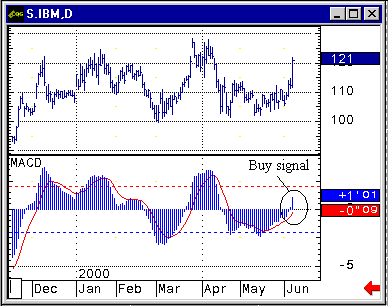HOT TOPICS LIST

INDICATORS LIST

LIST OF TOPICS

# Using The Moving Average Convergence/ Divergence (MACD) Indicator

06/12/00 11:13:20 AM
by Jason K. Hutson

Look at the chart for International Business Machines(IBM) There seems to be a buy signal created by using the MACD crossover.

Security:   IBM

 The moving average convergence/divergence (MACD) is a momentum oscillator developed by Gerald Appel in the early 1970s. The MACD measures the divergence or convergence of a shorter moving average with a longer moving average and is represented in two ways: a line form and a histogram. In this chart the histogram version is displayed. In the line version you will see two lines, the "fast" line which is the difference between the 12- and 26-period exponential moving average (EMA), and the "signal" line which is the EMA of the fast line.The histogram displays the difference between the fast and signal lines and are plotted above or below the zero line. It is the crossovers of the fast and signal lines, movement through the zero line, and divergences that generate the buy and sell signals. A sample code for calculating the MACD is:Calpha12 = 2/(12+1)Calpha26 = 2/(26+1)Calpha9 = 2/(9+1)Clast = last priceThe difference between the 12-period and 26-period exponetial moving averages has turned from a negative number to a positive number. This is shown above in the chart as "buy signal". Graphic provided by: CQG. *12 period exponetial moving averageEMA12N = Calpha12*(Clast) + (1-Calpha12)*Clastema12*26 period exponetial moving averageEMA26N = Calpha26*(Clast) + (1-Calpha26)*Clastema26MACDCDIFFMACD = EMA12N - EMA26NSignal LineEMAMACD = Calpha9*CDIFFMACD + (1-Calpha9)*Clastmacd

Jason K. Hutson

Traders.com Staff Writer. Enjoys trendlines, support and resistance, moving averages, RSI, MACD, ADX, Bollinger bands, parabolic SAR, chart formations, and volume analysis.

 Comments or Questions? Article Usefulness 5 (most useful) 4 3 2 1 (least useful)

Date:�/ /Rank:�1Comment:
Date:�/ /Rank:�3Comment:
Date:�/ /Rank:�3Comment:
Date:�/ /Rank:�4Comment: